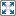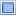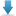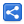# TypeScript - Using Interfaces to describe Indexable Types

[Last Updated: Sep 7, 2018]

In TypeScript, interfaces can also describe indexable types i.e. the types which can be accessed via indexes.

In JavaScript objects can be accessed via index. For example

```let x = {a: 1, b: 'blah'};
let n = x['a']; //same as x.a
let str = x['b']; // same as x.b```

In above snippet, x has properties with different value types. To force 'keys' to have same types and 'values' to have same types, TypeScript supports interfaces to describe indexable as reusable types.

Indexable types have an index signature that describes the types we can use to index into the object, along with the corresponding return types when indexing.

TypeScript only allows two types for indexes (the keys): string and number.

## Example

#### indexable-type-example.ts

```//defining reusable indexable type
interface States {
[state: string]: boolean;//indexer
}

let s: States = {'enabled': true, 'maximized':false};
console.log(s);
console.log(s['maximized']);```

#### Output

`{ enabled: true, maximized: false }false`

## TypeScript array vs positional indexer

Consider an example where we can index into the object by number type:

#### types-with-number-index.ts

```interface States {
[index: number]: boolean;
}

let s: States = [true, false];
console.log(s);
console.log(s);

```

#### Output

`[ true, false ]true`

As seen above, TypeScript supports array notation (the square brackets) to initialize positional indexable (arrays created with [] are also objects accessible via positional indexes).

Alternatively, we can use TypeScript arrays in above example:

#### using-array-example.ts

```let s: boolean[] = [true, false];
console.log(s);
console.log(s);```

#### Output

`[ true, false ]true`

So which one is better? The advantage of using interface to describe the 'number' indexable over using array is probably that the indexable interface, being a reusable type, can also be used for objects (created via {} notation). Also array has other members as well e.g. 'length', 'join()', 'push()', etc. The object created via indexer does not have them unless we also define those member in our interface (we will see an example on that shortly). So if we don't want to expose all array functionality we should use indexable types (with positional indexer).

## Supported index signature

As stated before, there are two types of supported index signatures: string and number. Following example attempts to use boolean:

#### wrong-indexable-type-example.ts

```interface boolArray {
[b: boolean]: string;
}

```

#### Output

`wrong-indexable-type-example.ts(2,6): error TS1023: An index signature parameter type must be 'string' or 'number'.`

## Indexer with other members

When using positional indexer (i.e. indexer of number type) we can define array properties/methods which need to be exposed.

#### with-members.ts

```interface States{
[index: number]: boolean;
length: number;
pop(): boolean;
}

let s: States = [true, false, true];
console.log(s);
console.log(s.length);
console.log(s.pop());
console.log(s);```

#### Output

`[ true, false, true ]3true[ true, false ]`

When using indexer of string type all properties should return the same type that the indexer returns

#### with-members2.ts

```interface States {
[state: string]: boolean;
mainScreen: boolean;
//following not allowed
//screenName: String;
}

let s: States = {mainScreen: false};
s["enabled"] = true;
s["maximized"] = false;
console.log(s);

```

#### Output

`{ mainScreen: false, enabled: true, maximized: false }`

If the indexer return type is 'any' then properties can return any types:

#### with-members3.ts

```interface States {
[state: string]: any;
screenName: String;
}

let s: States = {screenName:"main"};
s["enabled"] = true;
s["maximized"] = false;
s["width"]=300;
s["height"]=200;
console.log(s);

```

#### Output

`{ screenName: 'main',  enabled: true,  maximized: false,  width: 300,  height: 200 }`

## Example Project

Dependencies and Technologies Used:

• TypeScript 3.0.3
 Indexable TypesSelect AllDownload• typescript-indexable-types
• indexable-type-example.ts

 Share